# Elementary arithmetic functions in WPS Spreadsheet

Uploaded time: October 8, 2021 Difficulty: Beginner### Elementary arithmetic functions in WPS Spreadsheet

Tips: you can turn on the volume by clicking

Today I will introduce you to introductory calculation of functions, namely addition, subtraction, multiplication and division. In the table, there are three ways to enter the function, respectively, input in the formula bar input in the cell and click Insert Function.

So how can we do elementary arithmetic in the table? We can enter the corresponding symbol for the operation in the formula bar or cells. Take this table as an example, we enter = A2 + B2 in cell C2, press the Enter key to get the result.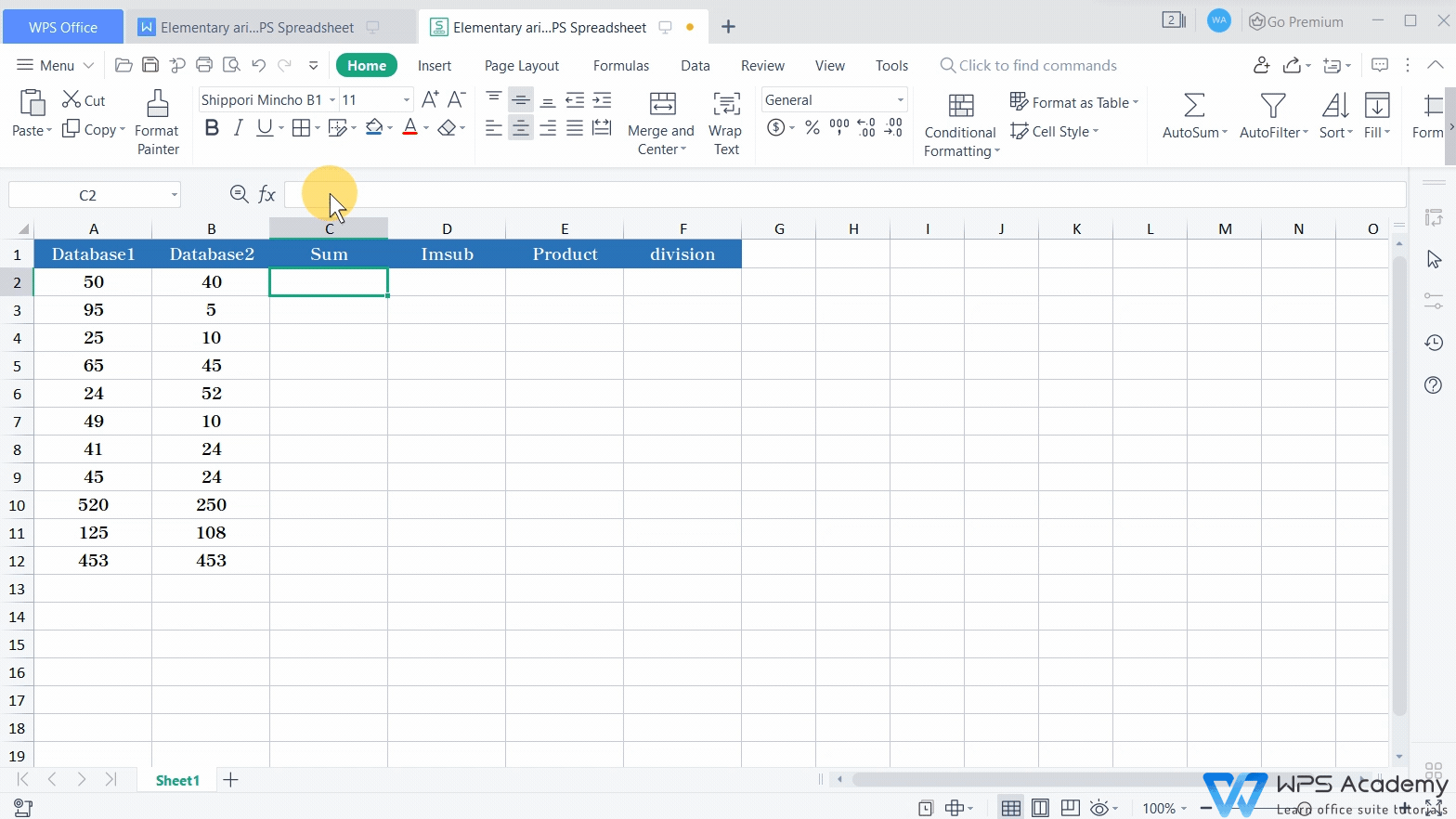Similarly, we select cell D2, enter =A2-B2, and press the Enter key to get the calculation results. Then, we select cell E2, enter“=A2*B2”, and press the Enter key to get the calculation results. The same goes for division. Select cell F2, enter“=A2/B2”, and press the Enter key to get the result.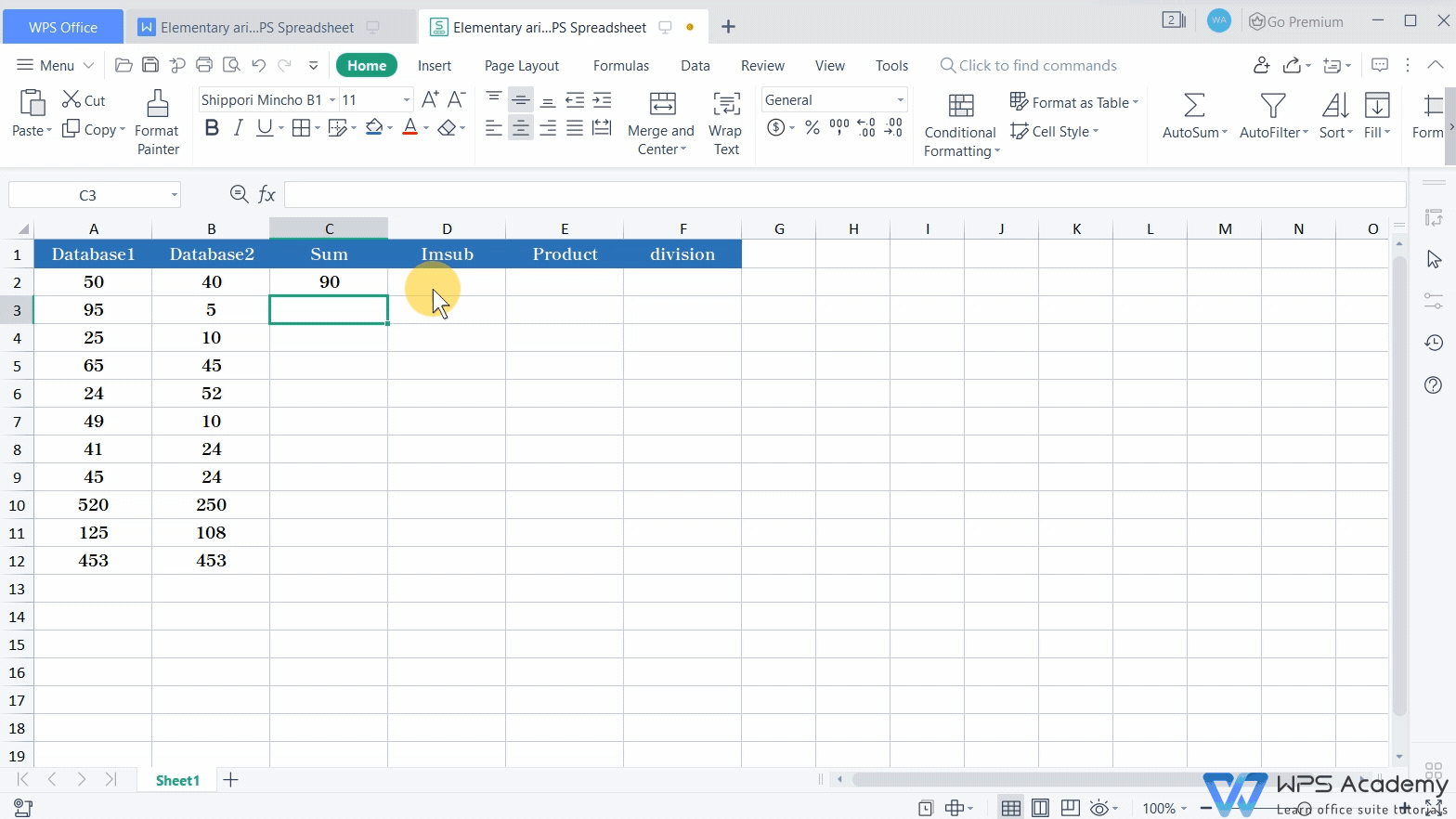Of course, we can also use the method of inserting function to do calculation. If we want to do the summation calculation, click the Formulas tab, and click the Insert Function button. In the popup dialog box, find the SUM function, enter the range of cells that needs to be summed, and click OK to quickly complete the summation.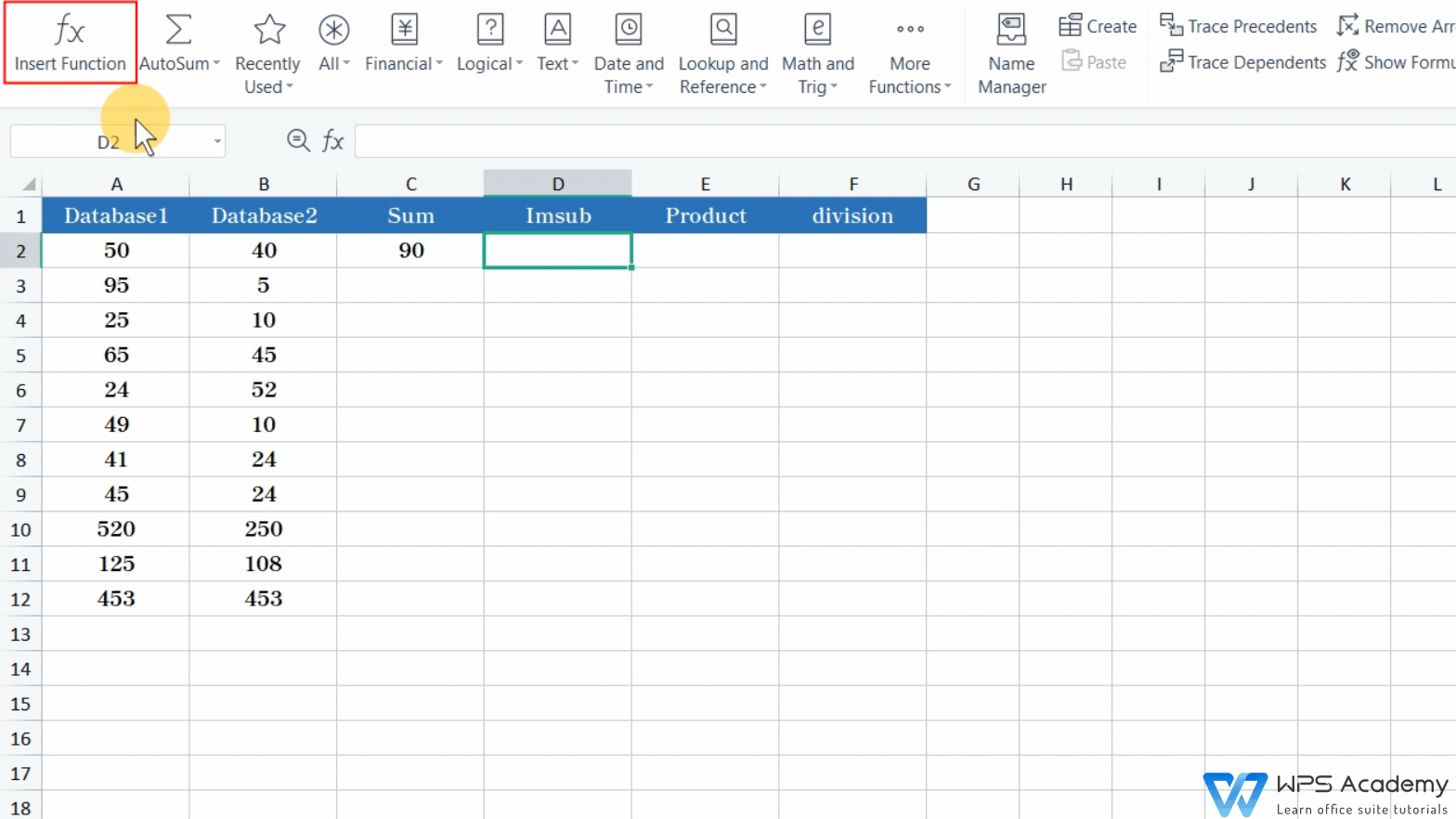If we want to do the subtraction calculation, select cell D2, open the Insert Function dialog box, find the IMSUB function, enter the range of cells that needs to be operated, and click OK to get the result.If we want to do the multiplication calculation, select cell E2, open the Insert Function dialog box, find the PRODUCT function, enter the range of cells that needs to be operated, and click OK to get the result.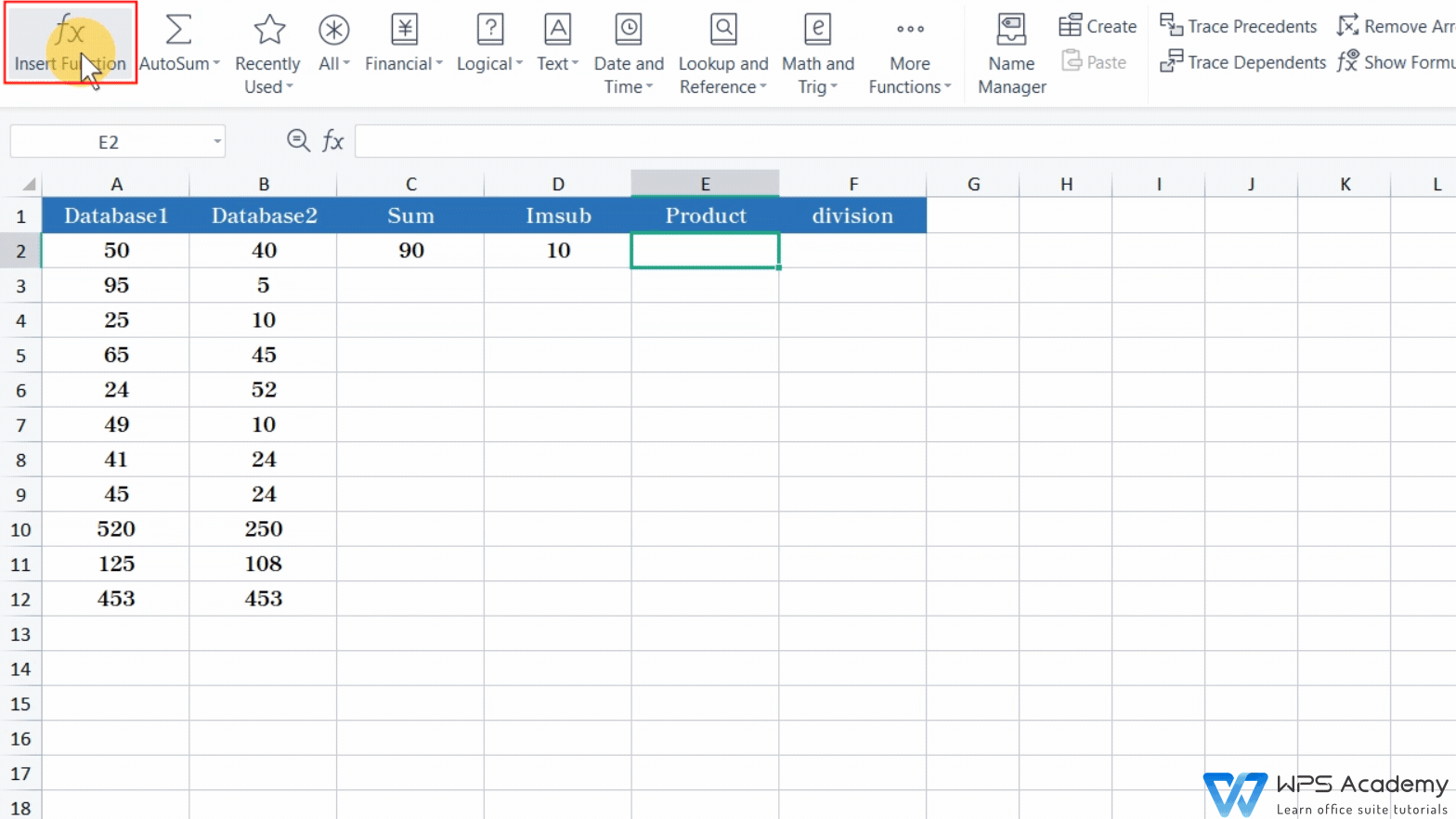There is no function for performing division calculation, but the division and multiplication are inverse operations. We can convert division into multiplication. Open the Insert Function dialog box, find the PRODUCT function, enter the range of cells that needs to be operated. Here we enter 1/B2 in the Number2 box, and click OK.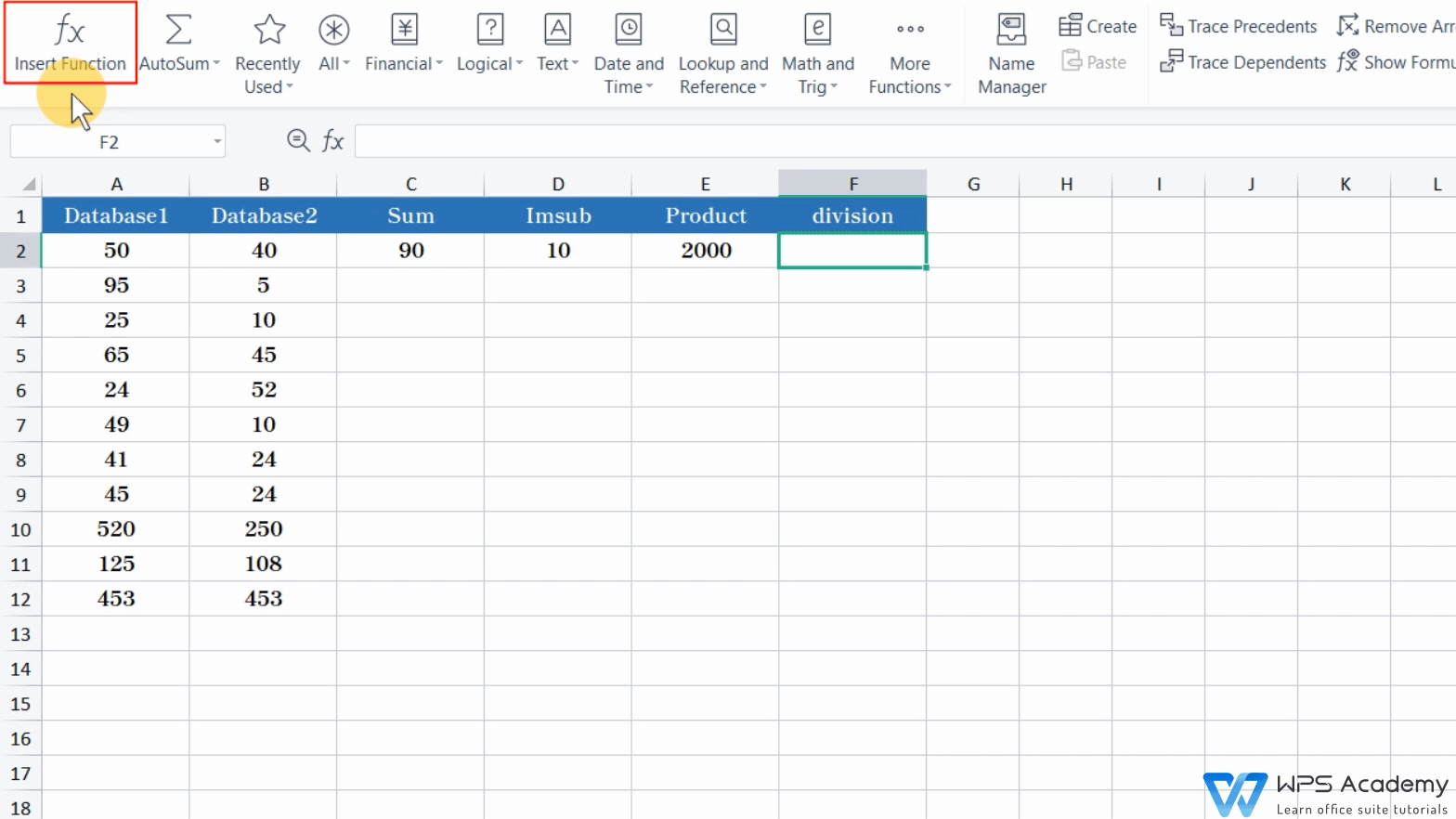Use the mouse to select cell B2:F2, double-click the fill handle to copy the formula, then we can quickly calculate the required data in batches.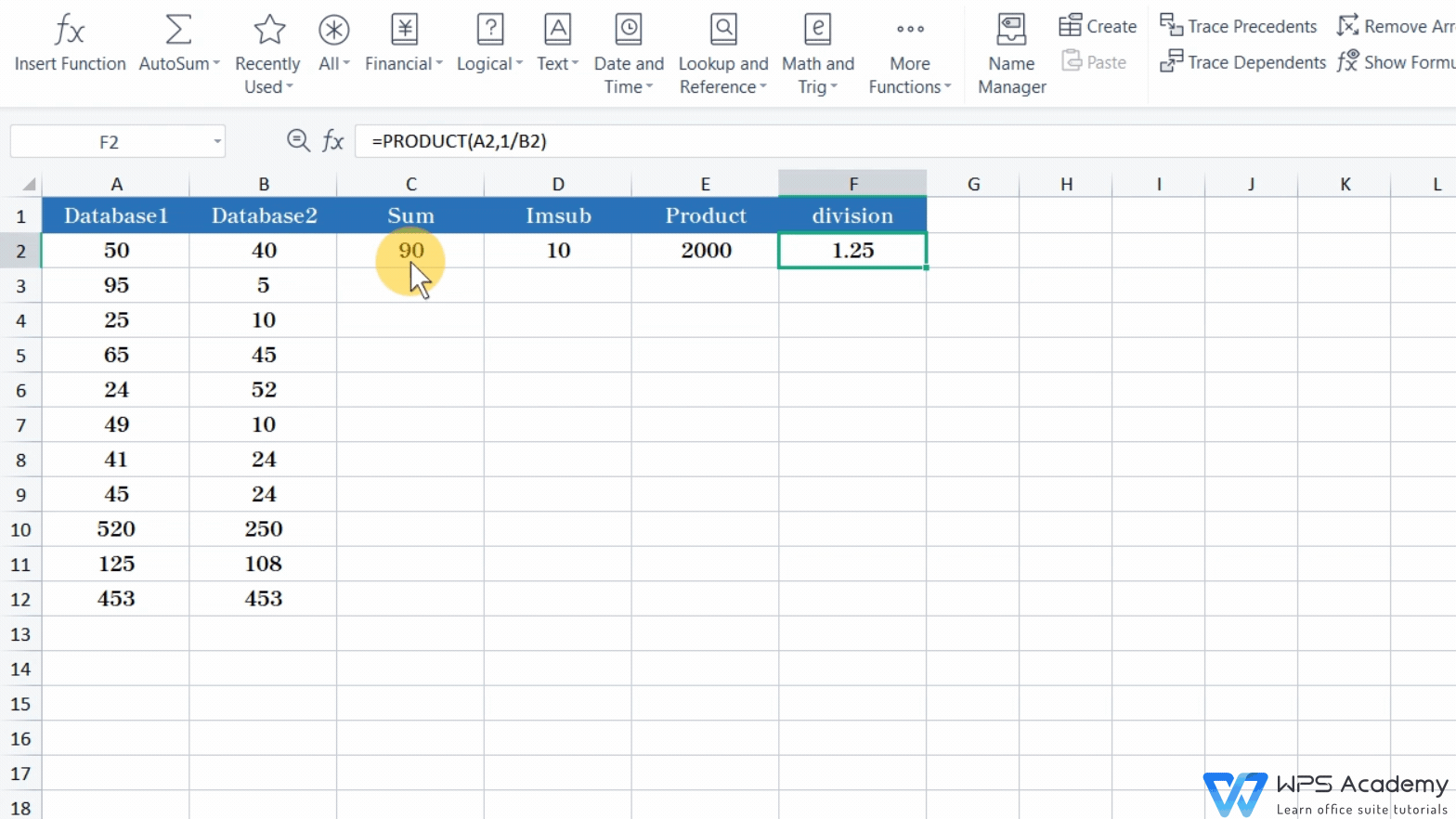Did you get it? To be office excel advanced, you could learn how to use WPS Office Spreadsheet online in WPS Academy.